SYSTEMS » UNIVERSAL MECHANISM » UM DRIVELINE

## UM DRIVE LINE

UM Driveline

Program package Universal Mechanism includes a specialized module UM Driveline, which contains force elements for simulation of drivelines e.g. in road, tracked, rail vehicles and other mechanical systems.DRIVE LINE

Mechanical rotation converter with a constant ratio

The element is used for simplified modeling a mechanical rotation converter with given transmission ratio i12 .In particular, the force element models gear trains, e.g. a gearbox.

Simplified model of a planetary drive

The force element is used for simplified modeling planetary gearing of four types shown in the figure. Simplification consists in neglecting the inertia properties of planet gears, i.e. it is assumed that kinetic energy of planets is small compared to energy of other parts and shafts. The model takes into account the compliance of teeth and bearings by introduction of an equivalent stiffness constant.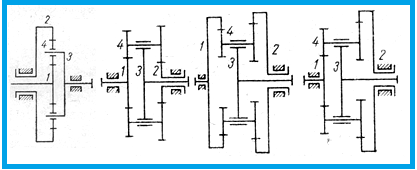PLANETARY TYPE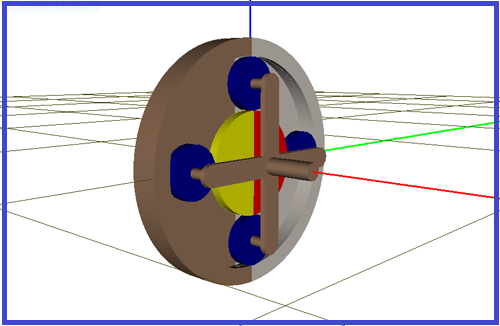PLANETARY DRIVE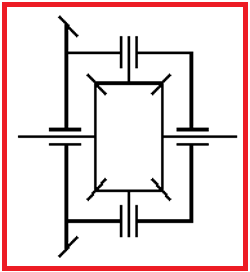DIFFERENTIAL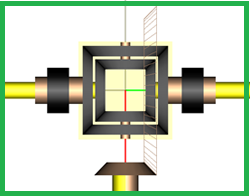DIFFERENTIAL UNIT

Differential

The differential is modeled as a force element connecting the input and axle shafts. The mathematical model of the differential is simplified by neglecting the inertia properties of the crown wheel, crown wheel housing and pinion gears, i.e. it is assumed that their contribution in kinetic energy is small. The model takes into account the compliance of side gears and pinion teeth as well as pinion bearings by introduction of an equivalent stiffness constant.

Fluid coupling

UM model of a fluid coupling is described by dependence of the torque coefficient on the transmission ratio.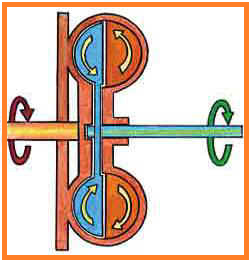FLUID COUPLING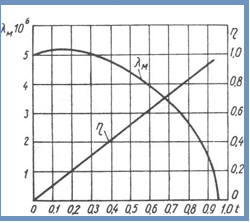FLUID COUPLING GRAPH

Hydraulic torque converter

The torque converter model can be described in several ways. Here are the equivalent converter description variants (the dependences of characteristics on the transmission ratio).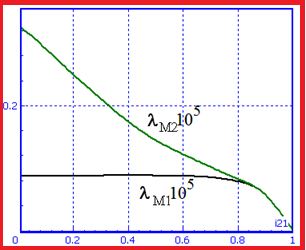TORQUE CONVERTER I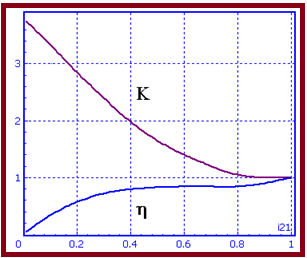TORQUE CONVERTER II

Hydrostatic drive

The following mathematical model of the hydrostatic drive is implemented: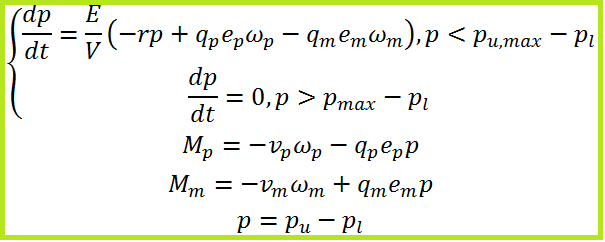HYDRO STATIC DRIVE I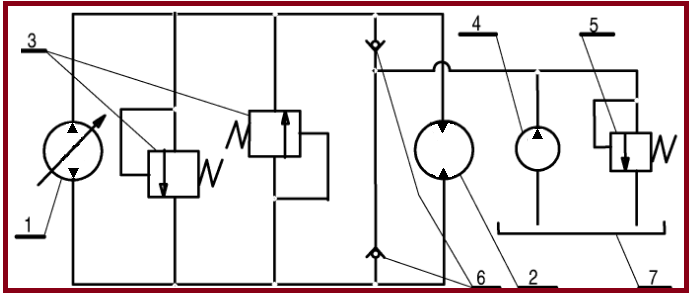HYDRO STATIC DRIVE II

Internal combustion engine

The UM model of internal combustion engine (ICE) is a torque Me нapplied to the engine crankshaft. The value of the torque depends on the shaft speed ne ∈ [nmin,nmax] , position of the throttle β ∈ [0,100%].

Me = Me(ne,β)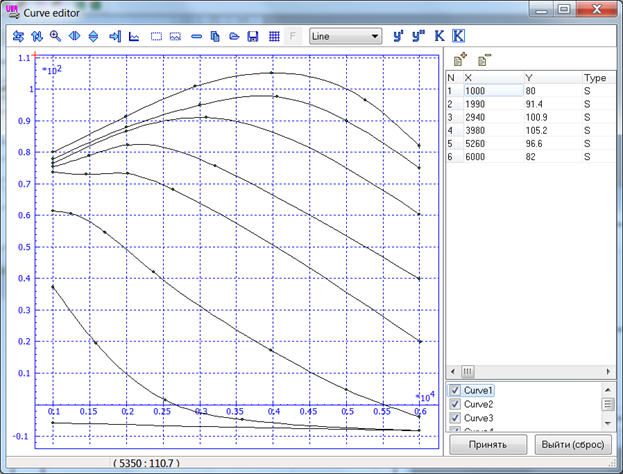Example pointwise engine map, β=100%, 79%, 76%, 49%, 37%, 24%, 12%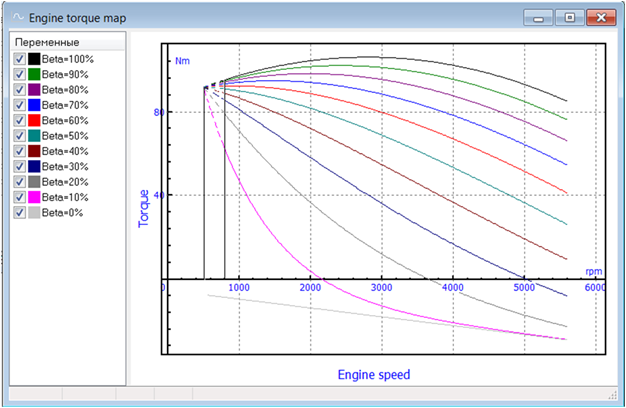Example of analytic engine map for spark ignition engine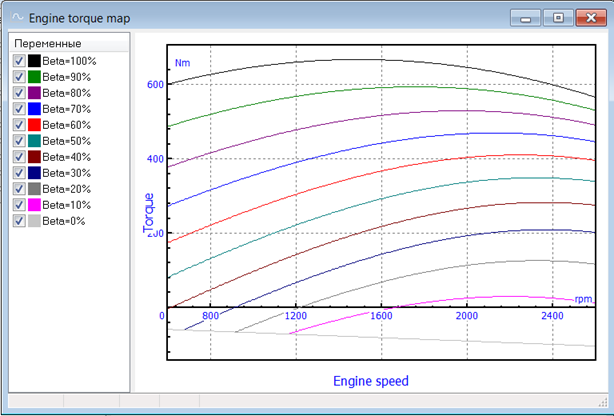Example: analytic engine map for diesel engine Kamaz 740.10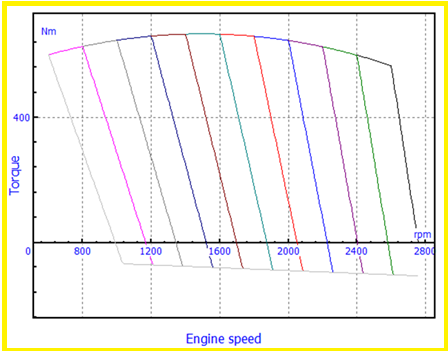Torque map for diesel engine with all-speed governor
info@paviathintegratedsolution.com www.paviathintegratedsolution.com